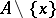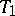Condensation point

(diff) ← Older revision | Latest revision (diff) | Newer revision → (diff)
The three notions mentioned above should be clearly distinguished. Ifis a subset of a topological spaceandis a point of, thenis an accumulation point ofif and only if every neighbourhood ofintersects. It is a condensation point ofif and only if every neighbourhood of it contains uncountably many points of.
The term limit point is slightly ambiguous. One might calla limit point ofif every neighbourhood ofcontains infinitely many points of, but this is not standard. Sometimes one callsa limit point of a net (cf. Net (of sets in a topological space)) if some subset of this net converges to. However, most people calla cluster point in this case.
In the case of a-spacethe notions of a limit point of a setand an accumulation point ofcoincide, and one uses "accumulation point" .